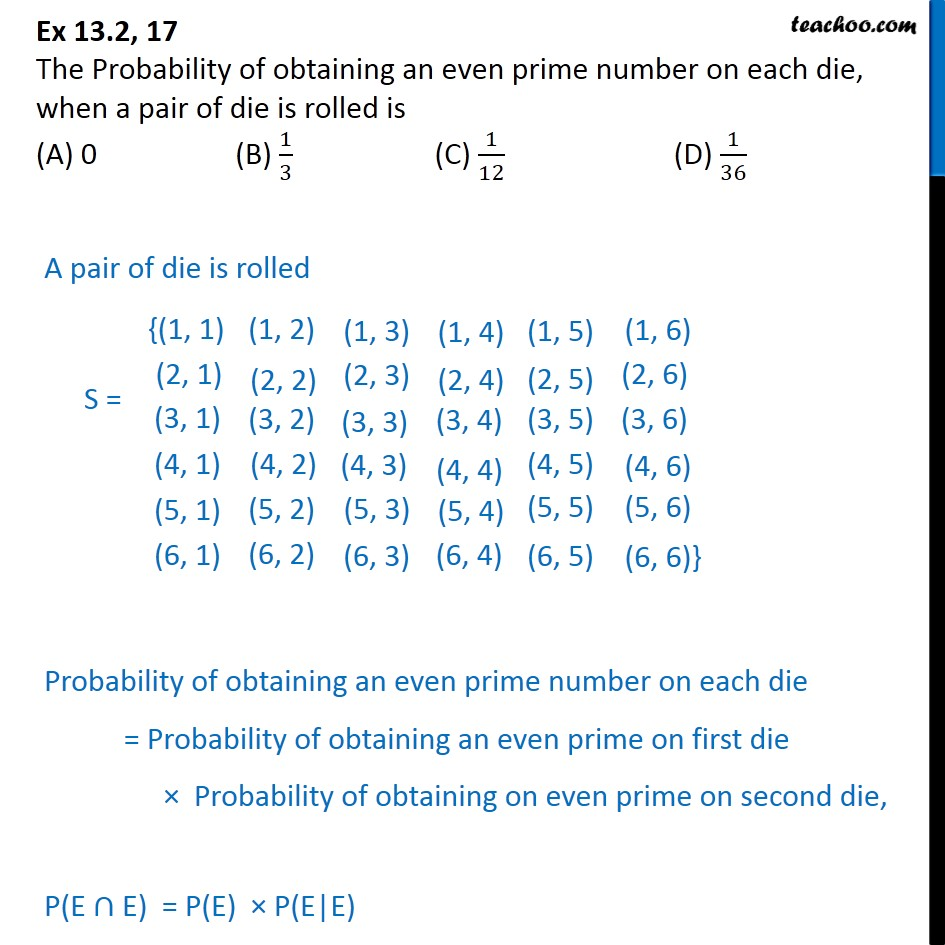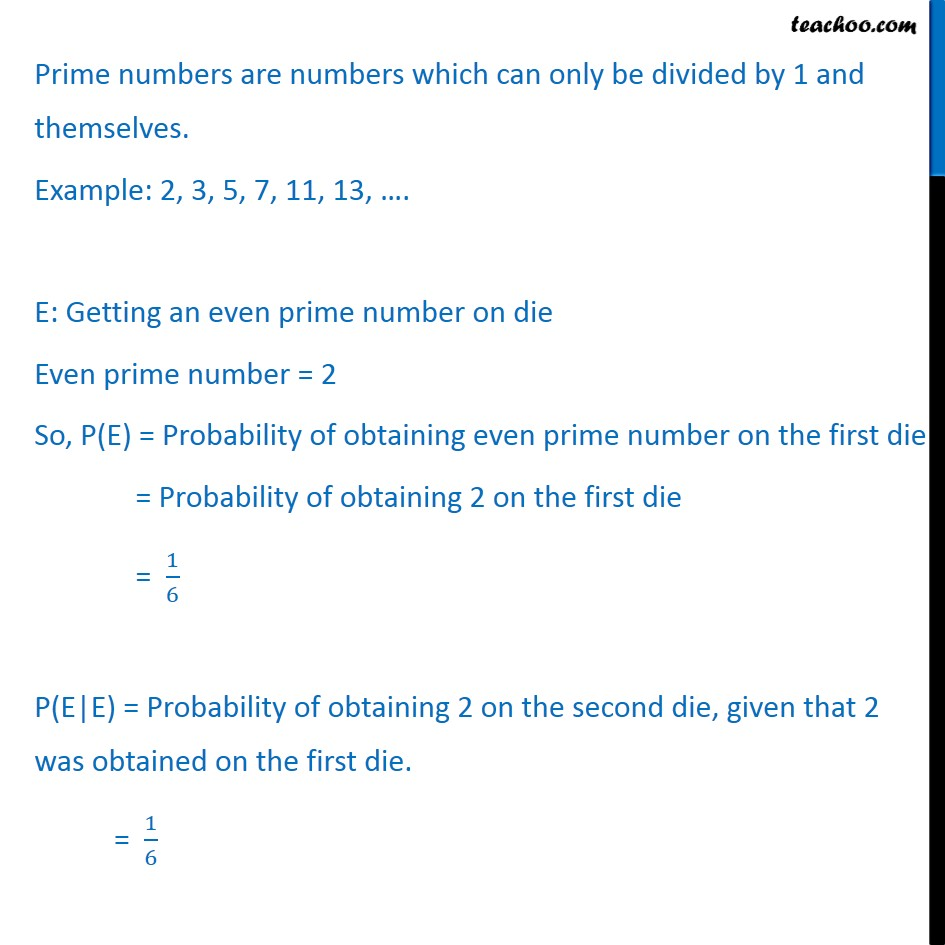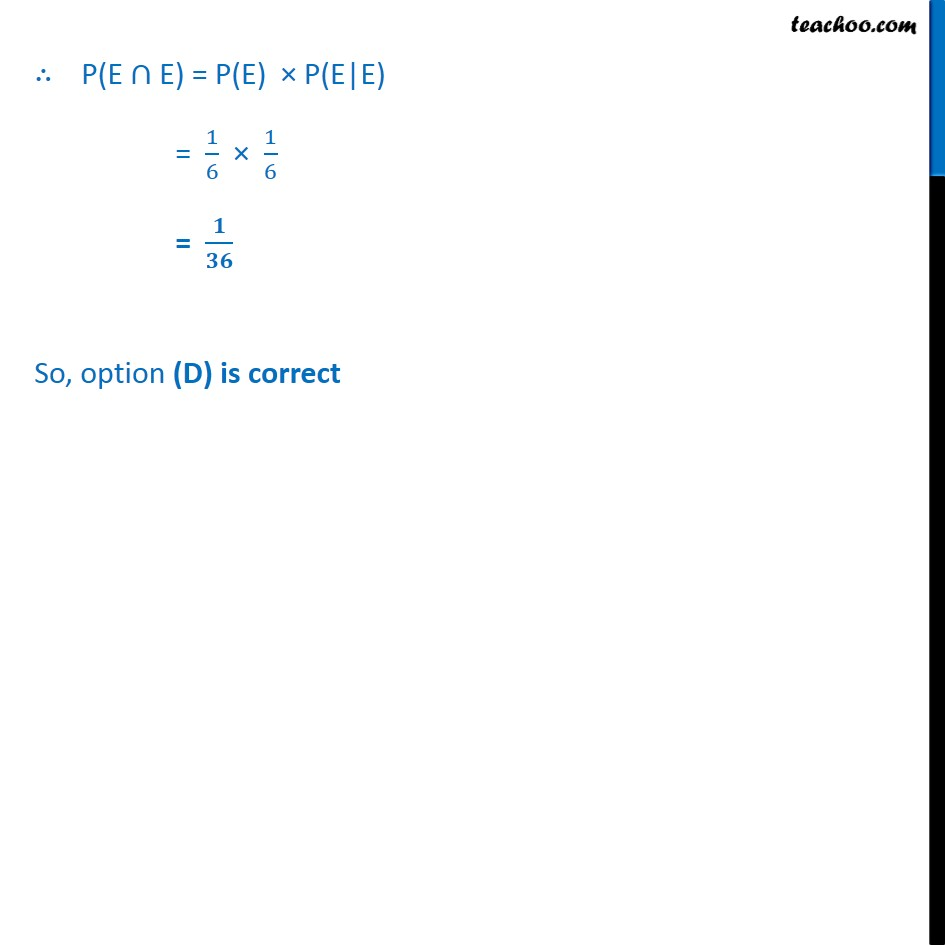1. Chapter 13 Class 12 Probability
2. Serial order wise
3. Ex 13.2

Transcript

Ex 13.2, 17 The Probability of obtaining an even prime number on each die, when a pair of die is rolled is (A) 0 (B) 1﷮3﷯ (C) 1﷮12﷯ (D) 1﷮36﷯ A pair of die is rolled S = Probability of obtaining an even prime number on each die = Probability of obtaining an even prime on first die × Probability of obtaining on even prime on second die, P(E ∩ E) = P(E) × P(E|E) Prime numbers are numbers which can only be divided by 1 and themselves. Example: 2, 3, 5, 7, 11, 13, …. E: Getting an even prime number on die Even prime number = 2 So, P(E) = Probability of obtaining even prime number on the first die = Probability of obtaining 2 on the first die = 1﷮6﷯ P(E|E) = Probability of obtaining 2 on the second die, given that 2 was obtained on the first die. = 1﷮6﷯ ∴ P(E ∩ E) = P(E) × P(E|E) = 1﷮6﷯ × 1﷮6﷯ = 𝟏﷮𝟑𝟔﷯ So, option (D) is correct

Ex 13.2